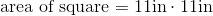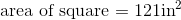ISEE Middle Level Math : Quadrilaterals

Example Questions

A square stamp has an edge length of 6 centimeters, what is its area?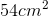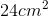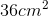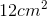Explanation:

A square stamp has an edge length of 6 centimeters, what is its area?

To find the area of a square, simply square the side length: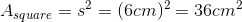If you have a square deck, what is the area when one of the sides isfeet?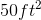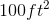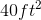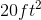Explanation:

The area of a square is the side length square, or multiplied by itself,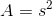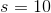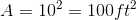.

A city block is a perfect square with sides of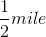.

What is the area of the city block?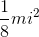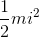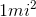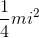Explanation:

A city block is a perfect square with sides of.

What is the area of the city block?

To find the area of a square, take the side length and square it.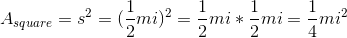So, we get:Find the area of a square with a base of length 13 inches.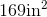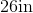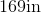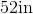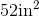Explanation:

To find the area of a square, we will use the following formula: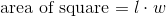where l is the length and w is the width of the square.

Now, we know the base of the square has a length of 13 inches.  Because it is a square, we know that all sides are equal.  Therefore, the width is also 13 inches.  Knowing this, we can substitute into the formula.  We get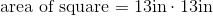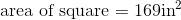Find the area of a square with a base of length 8 inches.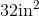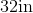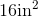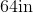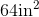Explanation:

To find the area of a square, we will use the following formula:where l is the length and w is the width of the square.

Now, we know the base has a length of 8 inches.  Because it is a square, we know that all sides are equal.  Therefore, all sides have a length of 8 inches.  This means the width is also 8 inches.  Knowing this, we can substitute into the formula.  We get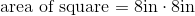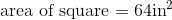Find the area of a square with a base of 12 inches.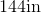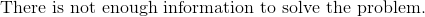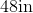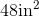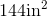Explanation:

To find the area of a square, we will use the following formula:where l is the length and w is the width of the square.

Now, we know the base of the square is 12in.  Because it is a square, we know that all sides are equal.  Which means all sides are 12in.  Therefore, the width is also 12in.  Knowing this, we can substitute into the formula.  We get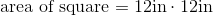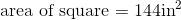Find the area of a square with a width of 5 feet.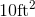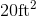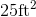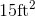Explanation:

To find the area of a square, we will use the following formula:where l is the length and w is the width of the rectangle.

Now, we know the width is 5 feet.  Because it is a square, all sides are equal.  This means the length is also 5 feet.

Knowing this, we can substitute into the formula.  We get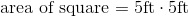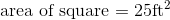Find the area of a square with a width of 12cm.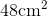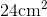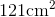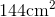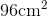Explanation:

To find the area of a square, we will use the following formula:where l is the length and w is the width of the square.

Now, we know the width of the square is 12cm.  Because it is a square, all sides are equal.  Therefore, the length is also 12cm.

Knowing this, we will substitute into the formula.  We get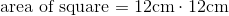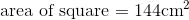Use the following image to answer the question: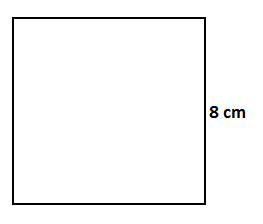Find the area of the square.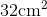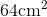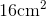Explanation:

To find the area of a square, we will use the following formula:where is the length and w is the width of the square.

Now, let's look at the given square.We can see the width is 8cm.  Because it is a square, we know that all sides are equal.  Therefore, the width is also 8cm.

Knowing this, we can substitute into the formula.  We get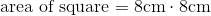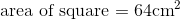Find the area of a square with a length of 11in.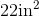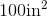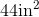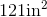Explanation:

To find the area of a square, we will use the following formula:where l is the length and w is the width of the square.

Now, we know the length of the square is 11in.  Because it is a square, all sides are equal.  Therefore, the width is also 11in.

Knowing this, we will substitute into the formula.  We get